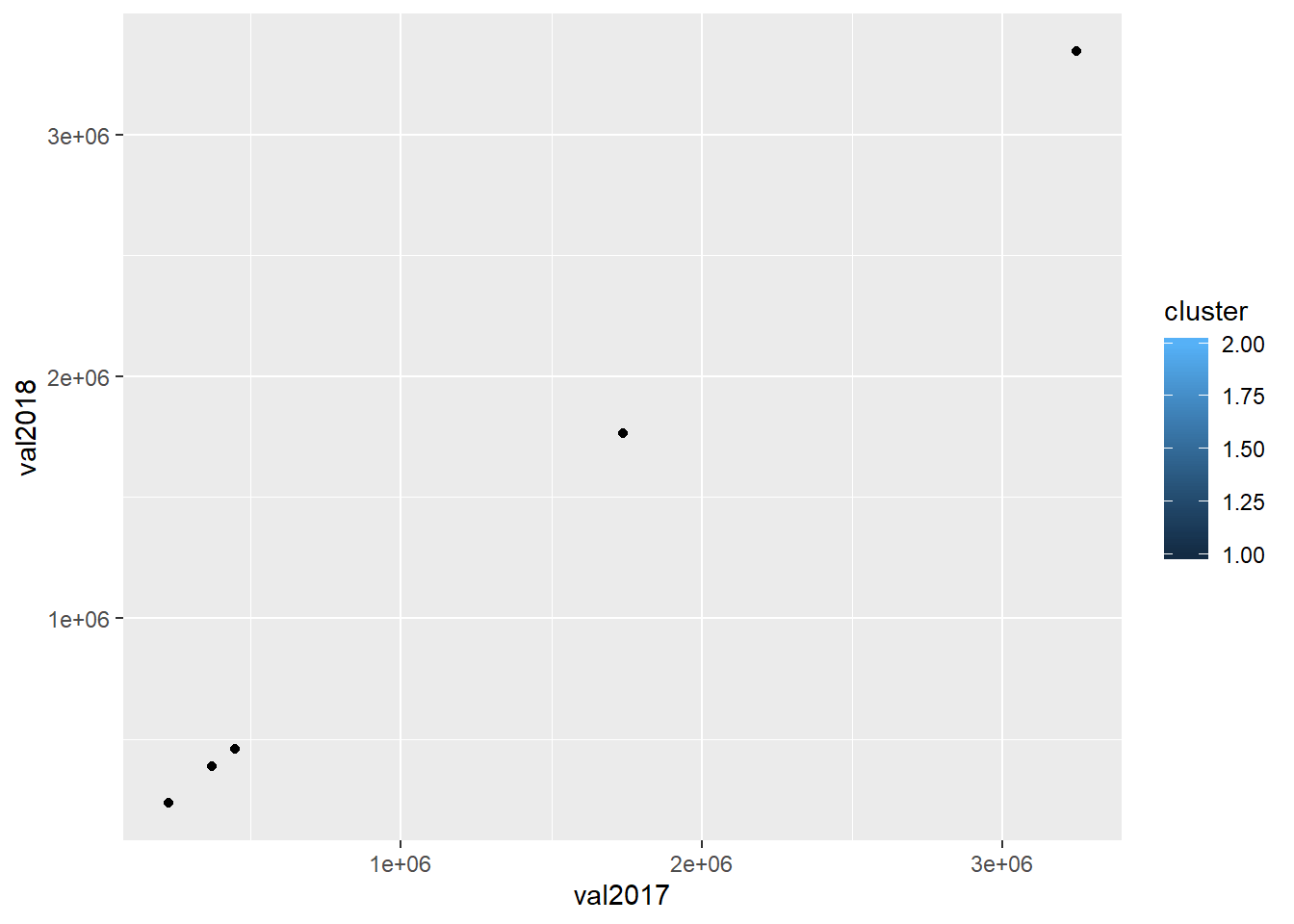# K-Means clustering performed on OECD data in RA small example demonstrating how to use dplyr in R for filtering retrieved OECD data (pulled via R interface using the OECD R-package)

In this post I show you: a) How to retrieve data from OECD database using OECD R-package, b) how to run k-means clustering in R using the base package, and c) how to visualize data with the ggplot2 R-package.

I will run a k-means clustering algorithm over a dataset retrieved from the OECD database, using the base and OECD packages in R.

First, I retrieve some OECD gdp data:

``````# import OECD package in R
library(OECD)

# retrieve economic gdp data measured with output methode - for all countries, between 2008 and 2019 ; for a country selection
# countries included:
# -- austria
# -- belgium
# -- finland
# -- germany
# -- italy

data_df <- as.data.frame(get_dataset(dataset = "SNA_TABLE1",
filter = list(c("AUT","BEL","FIN","DEU","ITA")),
start_time = 2017,
end_time = 2018))

# show the header of that dataset
``````##   LOCATION TRANSACT MEASURE TIME_FORMAT UNIT POWERCODE REFERENCEPERIOD obsTime
## 1      AUT    B1_GA       C         P1Y  EUR         6            <NA>    2017
## 2      AUT    B1_GA       C         P1Y  EUR         6            <NA>    2018
## 3      AUT B1G_P119       C         P1Y  EUR         6            <NA>    2017
## 4      AUT B1G_P119       C         P1Y  EUR         6            <NA>    2018
## 5      AUT    P3_P5       C         P1Y  EUR         6            <NA>    2017
## 6      AUT    P3_P5       C         P1Y  EUR         6            <NA>    2018
##   obsValue OBS_STATUS
## 1 370295.8       <NA>
## 2 385711.9       <NA>
## 3 330332.9       <NA>
## 4 344658.8       <NA>
## 5 357239.7       <NA>
## 6 371036.2       <NA>``````

## Processing the retrieved OECD dataset

In a next step I filter further, using dplyr:

``````# importing dplyr
library(dplyr)``````
``````library(magrittr)

# apply filter function from dplyr package
data_df <- data_df %>% filter(TRANSACT == "B1_GA",
MEASURE == "C",
TIME_FORMAT == "P1Y",
POWERCODE == "6")

# using base R functionality to ensure subsetting of dataset without any estimates or "ball-park-figures"
data_df <- data_df[is.na(data_df\$OBS_STATUS),]

# select columns relevant for further analysis
data_df <- data_df %>% select(LOCATION, obsTime, obsValue)

# sub-set into two different dataframes
data2017_df <- subset(data_df,obsTime == "2017")
data2018_df <- subset(data_df,obsTime == "2018")

# merge into one new data frame
joint_df <- inner_join(data2017_df,data2018_df,by="LOCATION") %>% select(LOCATION,obsValue.x,obsValue.y)
colnames(joint_df) <- c("country","val2017","val2018")

# view header of data subset
``````##   country   val2017   val2018
## 1     AUT  370295.8  385711.9
## 2     BEL  446364.9  459819.8
## 3     FIN  225785.0  234453.0
## 4     DEU 3244990.0 3344370.0
## 5     ITA 1736601.8 1765421.4``````

## Apply k-means clustering algorithm from R base package

Now I run a k-means clustering algorithm on this small dataset, using the base package in R. I will search for two cluster (middel) points, i.e. two centers:

``````# create cluster analysis object
clustering_obj <- kmeans(joint_df[,c(2,3)],centers=2)

# assign cluster to joint_df
joint_df\$cluster <- clustering_obj\$cluster``````

## Visualize results using the ggplot2 package in R

Using a colored scatterplot we can visualize cluster assignment in this small dataset:

``````# import ggplot2 library
library(ggplot2)

# create scatterplot grouped by cluster index, using discrete color scale
ggplot(joint_df) + geom_point(mapping = aes(x = val2017, y = val2018, fill = cluster))``````

If you want to learn more about the OECD R-package go ahead and check out my posts on how to retrieve e.g. inland freight OECD data in R.